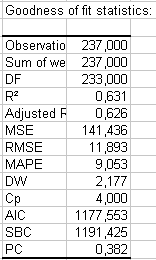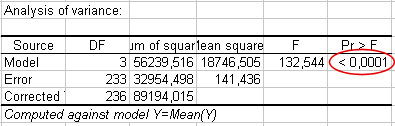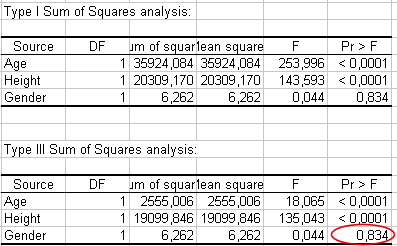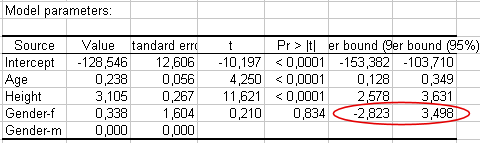Your data analysis solution

# ANCOVA analysis in Excel tutorial

2017-11-01

This tutorial will help you set up and interpret an Analysis of Covariance (ANCOVA) in Excel using the XLSTAT software.
Not sure this is the modeling feature you are looking for? Check out this guide.

## Dataset for running an ANCOVA

An Excel sheet with both the data and the results can be downloaded by clicking on the button below:

The data have been obtained in Lewis T. and Taylor L.R. (1967). Introduction to Experimental Ecology, New York: Academic Press, Inc. They concern 237 children, described by their Gender, Age in months, Height in inches (1 inch = 2.54 cm), and Weight in pounds (1 pound = 0.45 kg).

## Goal of this Analysis of Covariance (ANCOVA)

Using the Analysis of Covariance (ANCOVA), we want to find out how children weight varies with their gender (a qualitative variable that takes value form), their height and their age, and to verify if a linear model makes sense. The ANCOVA method belongs to a larger family of models called GLM (Generalized Linear Models) as do the linear regression and the ANOVA.

The specificity of ANCOVA is that it mixes qualitative and quantitative explanatory variables. In two other tutorials on linear regression, this dataset is also used, with the Height and then the Height and the Age as explanatory variables.

## Setting up an ANCOVA

After opening XLSTAT, select the XLSTAT / Modeling data / ANCOVA function.Once you've clicked on the button, the ANCOVA dialog box appears. Select the data on the Excel sheet. The Dependent variable (or variable to model) is here the Weight.

The quantitative explanatory variables are the height and the age. The qualitative variable is the gender. As we selected the column title for the variables, we leave the option Variable labels activated. The other options have been left at their default value.The computations begin once you have clicked on OK. The results will then be displayed.

## Interpreting the results of an ANCOVA

The first table displays the goodness of fit coefficients of the model. The R’² (coefficient of determination) indicates the % of the variability of the dependent variable which is explained by the explanatory variables. The closer to 1 the R’² is, the better the fit.In this particular case, 63 % of the variability of the Weight is explained by the Height, the Age, and the Gender. The remainder of the variability is due to some effects (other explanatory variables) that have not been or that could not be measured during this experiment. We can guess that some genetic and nutritive effects are involved, but it might be that simply by transforming the available variables we could obtain some better results.

It is important to examine the results of the analysis of variance table (see below). The results enable us to determine whether or not the explanatory variables bring significant information (null hypothesis H0) to the model. In other words, it's a way of asking yourself whether it is valid to use the mean to describe the whole population, or whether the information brought by the explanatory variables is of value or not.The Fisher's F test is used. Given the fact that the probability corresponding to the F value is lower than 0.0001, it means that we would be taking a lower than 0.01% risk in assuming that the null hypothesis (no effect of the two explanatory variables) is wrong. Therefore, we can conclude with confidence that the three variables do bring a significant amount of information.

We also want to find out if the three variables provide the same amount of information. To do this, we have to examine the Type I SS and Type III SS tables (see below). The Type I SS table is constructed by adding variables in the model one by one, and by evaluating the impact of each on the model sum of squares (Model SS). In consequence, in Type I SS, the order in which the variables are selected will influence the results. The lower the F probability corresponding to a given variable, the stronger the impact of the variable on the model as it is before the variable is added to it. We can see here that the Gender bring only a little information to the model, once the Height and the Age have been added.

The Type III SS table is computed by removing one variable of the model at a time to evaluate its impact on the quality of the model. This means that the order in which the variables are selected will not have any effect on the values in the Type III SS. The Type III SS is generally the best method to use to interpret results when an interaction is part of the model. The lower the F probability corresponding to a given variable, the stronger the impact of the variable on the model. We can see that the gender brings the least information to the model.The following table gives details on the model. This table is helpful when predictions are needed, or when you need to compare the coefficients of the model for a given population with the ones obtained for another population. We can see that the p-value for the Gender parameter is 0.83, and that the corresponding confidence range includes 0. This confirms the weak impact of the Gender on the model. If we look at the parameter corresponding to Gender-f, it seems that for a given age and height, being a girl means a small inccrease of the weight.The next table shows the residuals. It enables us to take a closer look at each of the standardized residuals. These residuals, given the assumptions of the linear regression model, should be normally distributed, meaning that 95% of the residuals should be in the interval [-1.96, 1.96]. All values outside this interval are potential outliers, or might suggest that the normality assumption is wrong. We used XLSTAT's DataFlagger (see the Tools toolbar) to bring out the residuals that are not in the [-1.96, 1.96] interval.

We can identify 16 suspicious residuals out of 237, that is to say, 6% instead of 5%, an analysis that could lead to rejecting the hypothesis of normality. A more in-depth analysis of the residuals has been performed in a tutorial on distribution fitting.

The chart below shows the predicted values versus the observed values. Confidence intervals allow to identify potential outliers.The residuals bar chart (see below) allows us to visualize the standardized residuals versus the Weight. It indicates that the residuals grow with the Weight. The histogram of the residuals enables us to quickly visualize the residuals that are out of the range [-2, 2].## Conclusion for this ANCOVA

As a conclusion, the Height, the Age, and the Gender allow us to explain 63% of the variability of the Weight. A significant amount of information is not explained by the ANCOVA model we have used. Further analyses would be necessary.

### Contact Us

#### Contact our technical support team: support@xlstat.com

https://cdn.desk.com/
false
desk
Loading
seconds ago
a minute ago
minutes ago
an hour ago
hours ago
a day ago
days ago
about
false
Invalid characters found
/customer/portal/articles/autocomplete
9283